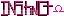-=+=- -=+=- -=+=- -=+=- -=+=- -=+=- -=+=- -=+=- -=+=- -=+=- -=+=- -=+=- -=+=- -=+=- -=+=- -=+=- -=+=- -=+=- -=+=- -=+=- -=+=- -=+=- -=+=- -=+=- -=+=- -=+=- -=+=- -=+=- -=+=- -=+=- (c) WidthPadding Industries 1987 0|203|0 -=+=- -=+=- -=+=- -=+=- -=+=- -=+=- -=+=- -=+=- -=+=- -=+=- -=+=- -=+=- -=+=- -=+=- -=+=- -=+=- -=+=- -=+=- -=+=- -=+=- -=+=- -=+=- -=+=- -=+=- -=+=- -=+=- -=+=- -=+=- -=+=- -=+=-
SoCoder -> Link Home -> Misc

StealthCreated : 10 July 2008
Edited : 10 July 2008

### Busted!

Iran + Photoshop ?!

https://littlegreenfootballs.com/article/30597_Irans_Photoshopped_Missile_Launch/
So it appears like Iran has been using Photoshop to make their pictures look good. Can you say busted?

Thursday, 10 July 2008, 23:16
JayenkaiHehe!
You might fool the world's press, but you can't fool the internet!Friday, 11 July 2008, 02:25
spinalI don't see how much more threatening four missiles is compared to three though.Friday, 11 July 2008, 03:25
moleI think it is more the fact only 3 successfully launched - one failed.

And they wouldn't want to show the Americans that they are incapable, would they...

I think it is pretty funny.Friday, 11 July 2008, 06:00
steve_ancellYeah, definitely a funny attempt of deception. But in my honest opinion, missiles are a disgrace to humanity and should not exist in the first place !.
Friday, 11 July 2008, 11:09
blanko1324Hah! That's pretty pathetic...Friday, 11 July 2008, 17:07
Schererererridiculous. that only makes them look even more stupid.